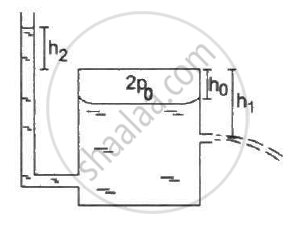Department of Pre-University Education, KarnatakaPUC Karnataka Science Class 11
Advertisement Remove all ads

# Figure Shows a Large Closed Cylindrical Tank Containing Water. Initially the Air Trapped Above the Water Surface Has a Height H0 and Pressure 2p0 Where P0 is the Atmospheric Pressure. - Physics

Sum

Figure shows a large closed cylindrical tank containing water. Initially the air trapped above the water surface has a height h0 and pressure 2p0 where p0 is the atmospheric pressure. There is a hole in the wall of the tank at a depth h1 below the top from which water comes out. A long vertical tube is connected as shown. (a) Find the height h2 of the water in the long tube above the top initially. (b) Find the speed with which water comes out of the hole. (c) Find the height of the water in the long tube above the top when the water stops coming out of the hole.Advertisement Remove all ads

#### Solution

(a) Pressure of water above the water level of the bigger tank is given by

P = (h_2 + h_0)rhog

Let the atmospheric pressure above the tube be P_0.

Total pressure above the tube = P_0 + P

=(h_2+h_0)rhog+P_0

This pressure initially is balanced by pressure above the tank 2P_0.

⇒ 2P_0 = (h_2 + h_0)rhog + P_0

⇒ h_2 = (P_0)/(rhog) - h_0

(b) Velocity of the efflux out of the outlet depends upon the total pressure above the outlet.Total pressure above the outlet = 2P_0 + (h_1 - h_0)rhog

Applying Bernouli's law, we get

Let the velocity of efflux be v1 and the velocity with which the level of the tank falls be v2 . pressure above the outlet is P_0. Then,

(2P_0+(h_1-h_0)rhog)/rho + gz + v_2^2/2 = P_0/rho+gz+v_2^2/2

Now , let the reference point of the liquid be the level of the outlet . Thus, z = 0

⇒ (P_0+(h_1 - h_0)rhog)/(rho) + v_2^2/2 = v_1^2/2

Again , the speed with which the water level of the tank goes down is very less compared to the velocity of the efflux . Thus,

"v"_2 = 0

⇒ (P_0+(h_1-h_0)rhog)/(rho) = v_1^2/2

⇒ v_1 = [2/rho(P_0 + (h_1 - h_0) rhog)]^(1/2)

(c) Water maintains its own level , so height of the water of the tank will be "h"_1 when water will stop flowing

Thus height of water in the tube below the tank height will be = h1

Hence height of the water above the tank height will be = -h1

Concept: Kinetic Theory of Gases- Assumptions
Is there an error in this question or solution?
Advertisement Remove all ads

#### APPEARS IN

HC Verma Class 11, Class 12 Concepts of Physics Vol. 2
Chapter 2 Kinetic Theory of Gases
Q 42 | Page 36
Advertisement Remove all ads

#### Video TutorialsVIEW ALL 

Advertisement Remove all ads
Share
Notifications

View all notifications

Forgot password?